# Poker Hand Detection¶

Database Creatores:
Robert Cattral (cattral@gmail.com)
Franz Oppacher (oppacher@scs.carleton.ca)
Carleton University, Department of Computer Science Intelligent Systems Research Unit
1125 Colonel By Drive, Ottawa, Ontario, Canada, K1S5B6
https://archive.ics.uci.edu/ml/datasets/Poker+Hand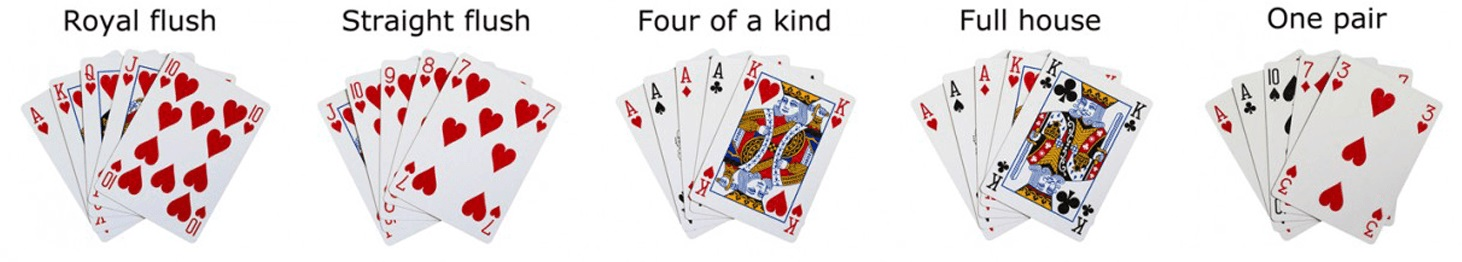The Poker Hand database consists of 1,025,010 instances of poker hands. Each instance is an example of a poker hand consisting of five cards drawn from a standard deck of 52 cards. Each card is described using two attributes (suit and rank), for a total of 10 features. There is one Class attribute that describes the Poker Hand. The order of cards is important, which is why there are 480 possible Royal Flush hands as compared to 4 (one for each suit  explained in more detail below).

## Feature Information¶

1. S1 - Suit of card 1
 Ordinal (1-4) representing: Hearts=1, Spades=2, Diamonds=3, Clubs=4

 1 heart ♥ 2 spade ♠ 3 diamond ♦ 4 club ♣

2. C1 - Rank of card 1
 Numerical (1-13) representing: Ace=1, 2, 3, 4, 5, 6, 7, 8, 9, 10 , Jack=11, Queen=12, King=13

3. S2 - Suit of card 2
 Ordinal (1-4) representing: Hearts=1, Spades=2, Diamonds=3, Clubs=4

4. C2 - Rank of card 2
 Numerical (1-13) representing: Ace=1, 2, 3, 4, 5, 6, 7, 8, 9, 10 , Jack=11, Queen=12, King=13

5. S3 - Suit of card 3
 Ordinal (1-4) representing: Hearts=1, Spades=2, Diamonds=3, Clubs=4

6. C3 - Rank of card 3
 Numerical (1-13) representing: Ace=1, 2, 3, 4, 5, 6, 7, 8, 9, 10 , Jack=11, Queen=12, King=13

7. S4 - Suit of card 4
 Ordinal (1-4) representing: Hearts=1, Spades=2, Diamonds=3, Clubs=4

8. C4 - Rank of card 4
 Numerical (1-13) representing: Ace=1, 2, 3, 4, 5, 6, 7, 8, 9, 10 , Jack=11, Queen=12, King=13

9. S5 - Suit of card 5
 Ordinal (1-4) representing: Hearts=1, Spades=2, Diamonds=3, Clubs=4

10. C5 - Rank of card 5
 Numerical (1-13) representing: Ace=1, 2, 3, 4, 5, 6, 7, 8, 9, 10 , Jack=11, Queen=12, King=13

11. CLASS Poker Hand Ordinal (0-9)

0 - Nothing in hand; not a recognized poker hand
1 - One pair; one pair of equal ranks within five cards
2 - Two pairs; two pairs of equal ranks within five cards
3 - Three of a kind; three equal ranks within five cards
4 - Straight; five cards, sequentially ranked with no gaps
5 - Flush; five cards with the same suit
6 - Full house; pair + different rank three of a kind
7 - Four of a kind; four equal ranks within five cards
8 - Straight flush; straight + flush
9 - Royal flush; {Ace, King, Queen, Jack, Ten} + flush


### Example¶

The following poker hand will be represented in the database by the following 10 features vector
1, 1, 4, 1, 2, 10, 3, 7, 1, 3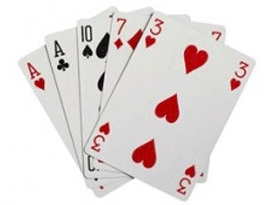## Prerequisites¶

To run the code below you'll need to download following private libraries which we use in several examples of this course:

https://github.com/samyzaf/kerutils

In :
import pandas as pd
import matplotlib.pyplot as plt
from matplotlib import rcParams
rcParams['figure.figsize'] = 10,7

# Our deep learning library is Keras
from keras.models import Sequential
from keras.layers.core import Dense, Dropout
from keras.utils.np_utils import to_categorical
import numpy as np
# fixed random seed for reproducibility
np.random.seed(0)
# private libraies
from kerutils import *
%matplotlib inline

Using Theano backend.
DEBUG: nvcc STDOUT mod.cu
Creating library C:/Users/samy/AppData/Local/Theano/compiledir_Windows-10-10.0.14393-Intel64_Family_6_Model_94_Stepping_3_GenuineIntel-2.7.11-64/tmpq23sba/265abc51f7c376c224983485238ff1a5.lib and object C:/Users/samy/AppData/Local/Theano/compiledir_Windows-10-10.0.14393-Intel64_Family_6_Model_94_Stepping_3_GenuineIntel-2.7.11-64/tmpq23sba/265abc51f7c376c224983485238ff1a5.exp

Using gpu device 0: GeForce GTX 950 (CNMeM is enabled with initial size: 80.0% of memory, cuDNN 5103)
c:\anaconda2\lib\site-packages\theano\sandbox\cuda\__init__.py:600: UserWarning: Your cuDNN version is more recent than the one Theano officially supports. If you see any problems, try updating Theano or downgrading cuDNN to version 5.
warnings.warn(warn)

In :
# These are css/html styles for good looking ipython notebooks
from IPython.core.display import HTML
HTML('<style>{}</style>'.format(css))

Out:
In :
features = ['S1', 'C1', 'S2', 'C2', 'S3', 'C3', 'S4', 'C4', 'S5', 'C5', 'CLASS']

In :
# Lets view the first 10 rows of the data set
# See bellow what these names mean


Out:
S1 C1 S2 C2 S3 C3 S4 C4 S5 C5 CLASS
0 1 10 1 11 1 13 1 12 1 1 9
1 2 11 2 13 2 10 2 12 2 1 9
2 3 12 3 11 3 13 3 10 3 1 9
3 4 10 4 11 4 1 4 13 4 12 9
4 4 1 4 13 4 12 4 11 4 10 9
5 1 2 1 4 1 5 1 3 1 6 8
6 1 9 1 12 1 10 1 11 1 13 8
7 2 1 2 2 2 3 2 4 2 5 8
8 3 5 3 6 3 9 3 7 3 8 8
9 4 1 4 4 4 2 4 3 4 5 8
In :
# How many rows do we have?

len(data.index)

Out:
25010
In :
# How many columns do we have?

len(data.columns)

Out:
11
In :
# How many records do we have in our data set?

data.size    # 11 * 25010

Out:
275110
In :
# You can get everything in one line !

data.shape

Out:
(25010, 11)
In :
# View the last 10 records of our data set

data.tail(10)

Out:
S1 C1 S2 C2 S3 C3 S4 C4 S5 C5 CLASS
25000 2 8 2 12 4 3 4 2 4 4 0
25001 2 12 3 5 3 8 4 1 4 2 0
25002 4 10 2 13 4 5 4 7 1 5 1
25003 1 12 2 9 2 12 4 8 1 13 1
25004 3 5 3 7 4 11 3 11 3 2 1
25005 3 9 2 6 4 11 4 12 2 4 0
25006 4 1 4 10 3 13 3 4 1 10 1
25007 2 1 2 10 4 4 4 1 4 13 1
25008 2 12 4 3 1 10 1 12 4 9 1
25009 1 7 3 11 3 3 4 8 3 7 1
In :
# Some statistics to get acquainted with the data

nb_classes = 10  # we have 10 classes of poker hands
cls = {}
for i in range(nb_classes):
cls[i] = len(data[data.CLASS==i])
print(cls)

{0: 12493, 1: 10599, 2: 1206, 3: 513, 4: 93, 5: 54, 6: 36, 7: 6, 8: 5, 9: 5}

In :
# Let's keep a map of poker hand class id to class name
poker_hands = [0, 1, 2, 3, 4, 5, 6, 7, 8, 9]
hand_name = {
0: 'Nothing in hand',
1: 'One pair',
2: 'Two pairs',
3: 'Three of a kind',
4: 'Straight',
5: 'Flush',
6: 'Full house',
7: 'Four of a kind',
8: 'Straight flush',
9: 'Royal flush',
}

In :
for i in poker_hands:
print("%s: %d" % (hand_name[i], cls[i]))

Nothing in hand: 12493
One pair: 10599
Two pairs: 1206
Three of a kind: 513
Straight: 93
Flush: 54
Full house: 36
Four of a kind: 6
Straight flush: 5
Royal flush: 5


The classes are highly imbalanced, which could hamper the training process. Our deep learning model will learn a lot about "One Pair" (10599 hands) but very little about "Royal Flash" (only 5 hands) !?

It is usually a good practice to keep this in mind and draw a class distribution bar chart before you start building and training deep learning models. The bar chart will give you a thick visual clue regarding imbalance.

In :
plt.bar(poker_hands, [cls[i] for i in poker_hands], align='center')
plt.xlabel('Poker hand id')
plt.ylabel('Number of instances')

Out:
<matplotlib.text.Text at 0x6314d6d8>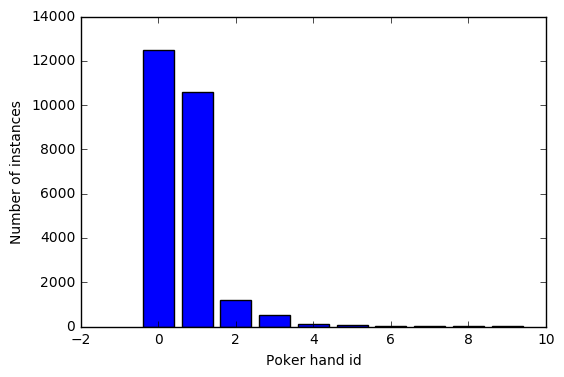### Deep Learning with Keras¶

We now proceed to build a neural network using Keras for detecting the hand poker class from the 10 feature of the 5 cards at hand. We will first split our data to the first 10 predictive features and the last CLASS feature will be converted to a catogorical form. As usuall, Pandas DataFrames must be converted to Numpy matrices in order to be recognized by Keras.

In :
#  Let's first extract the first 10 features from our data (from the 11 we have)
#  We want to be able to predict the class (hand poker type)

X_train = data.iloc[:,0:10].as_matrix()
y_train = data.iloc[:,10].as_matrix()

In :
# let's look at the first 20 records sample

X_train[0:20]

Out:
array([[ 1, 10,  1, 11,  1, 13,  1, 12,  1,  1],
[ 2, 11,  2, 13,  2, 10,  2, 12,  2,  1],
[ 3, 12,  3, 11,  3, 13,  3, 10,  3,  1],
[ 4, 10,  4, 11,  4,  1,  4, 13,  4, 12],
[ 4,  1,  4, 13,  4, 12,  4, 11,  4, 10],
[ 1,  2,  1,  4,  1,  5,  1,  3,  1,  6],
[ 1,  9,  1, 12,  1, 10,  1, 11,  1, 13],
[ 2,  1,  2,  2,  2,  3,  2,  4,  2,  5],
[ 3,  5,  3,  6,  3,  9,  3,  7,  3,  8],
[ 4,  1,  4,  4,  4,  2,  4,  3,  4,  5],
[ 1,  1,  2,  1,  3,  9,  1,  5,  2,  3],
[ 2,  6,  2,  1,  4, 13,  2,  4,  4,  9],
[ 1, 10,  4,  6,  1,  2,  1,  1,  3,  8],
[ 2, 13,  2,  1,  4,  4,  1,  5,  2, 11],
[ 3,  8,  4, 12,  3,  9,  4,  2,  3,  2],
[ 1,  3,  4,  7,  1,  5,  2,  4,  4, 13],
[ 1,  4,  1,  1,  1,  3,  3,  5,  3,  2],
[ 3,  8,  3, 12,  2,  7,  2,  6,  1,  2],
[ 4,  8,  1, 11,  4,  6,  3,  2,  4, 12],
[ 3,  7,  2,  7,  4, 11,  1, 12,  3,  1]], dtype=int64)
In :
y_train[0:20]

Out:
array([9, 9, 9, 9, 9, 8, 8, 8, 8, 8, 1, 0, 0, 0, 1, 0, 4, 0, 0, 1], dtype=int64)

## Keras Model Defintion¶

1. An input layer of 10 neurons
2. A hidden layer of 16 neurons
3. An ouput layer of 10 neurons (one for each poker hand class)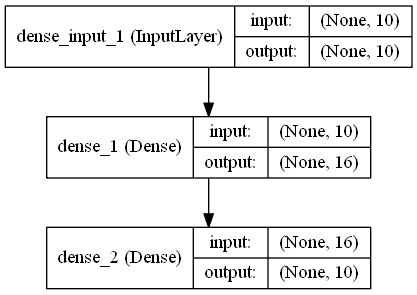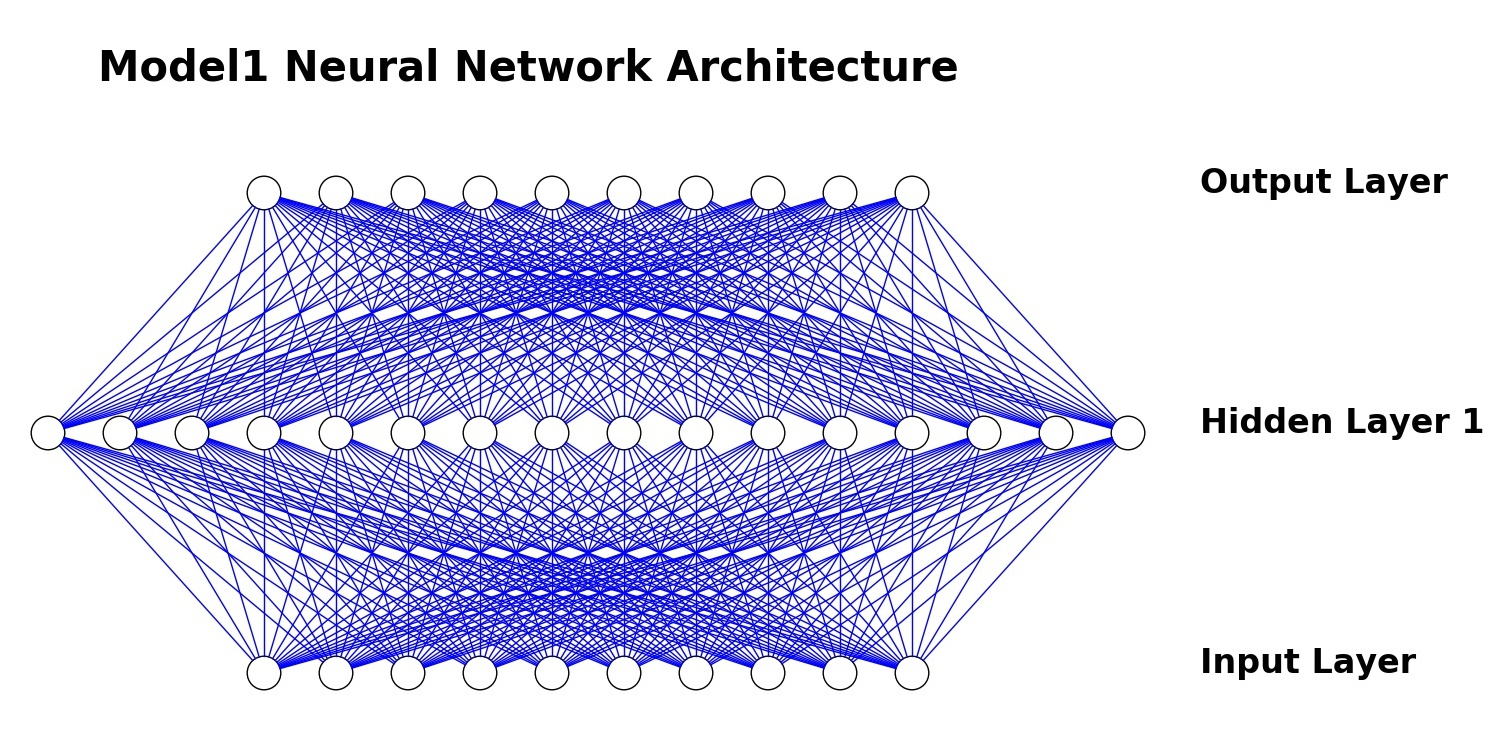In :
# Our first Keras Model
model1 = Sequential()


### Compiling the model¶

In :
model1.compile(loss='categorical_crossentropy', optimizer='adam', metrics=['accuracy'])


## Training our Model¶

Keras training method (fit) expects one-hot binary vectors as class output. Hence Keras contains a special utility (to_categorical) for converting integer classes to one-hot binary vectors. One-hot form conversion looks like this:

0 -> [1, 0, 0, 0, 0, 0, 0, 0, 0, 0]
1 -> [0, 1, 0, 0, 0, 0, 0, 0, 0, 0]
2 -> [0, 0, 1, 0, 0, 0, 0, 0, 0, 0]
etc.

In :
Y_train = to_categorical(y_train)


We will use 300 epochs and a batch_size of 32. We also use our FitMonitor callback from our kerutils library (see above download links), which is a more compact progress monitor with intermediate summaries after each 10% period. The view_acc utility is useful for viewing the model training accuracy.

In :
# We use our FitMonitor callback from our kerutils library (see above for download)
fmon = FitMonitor()
h = model1.fit(X_train, Y_train, nb_epoch=300, batch_size=32, verbose=0, callbacks=[fmon])
# h is a history object that records the fitting process

Train begin: 2016-11-20 15:03:07
batch_size = 32
do_validation = False
metrics = ['loss', 'acc']
nb_epoch = 300
nb_sample = 25010
verbose = 0
.......... 10% epoch=30 acc=0.579528 loss=0.896328 max_acc=0.580768 max_val_acc=-1.000000
.......... 20% epoch=60 acc=0.577009 loss=0.898772 max_acc=0.580768 max_val_acc=-1.000000
.......... 30% epoch=90 acc=0.577369 loss=0.895959 max_acc=0.581687 max_val_acc=-1.000000
.......... 40% epoch=120 acc=0.577649 loss=0.896047 max_acc=0.581687 max_val_acc=-1.000000
.......... 50% epoch=150 acc=0.580688 loss=0.894722 max_acc=0.581687 max_val_acc=-1.000000
.......... 60% epoch=180 acc=0.577809 loss=0.897578 max_acc=0.581687 max_val_acc=-1.000000
.......... 70% epoch=210 acc=0.578289 loss=0.895841 max_acc=0.581687 max_val_acc=-1.000000
.......... 80% epoch=240 acc=0.578968 loss=0.894176 max_acc=0.581887 max_val_acc=-1.000000
.......... 90% epoch=270 acc=0.576409 loss=0.897671 max_acc=0.581887 max_val_acc=-1.000000
......... 99% epoch=299 acc=0.576889 loss=0.899985
Train end: 2016-11-20 15:07:57
Total run time: 290.03 seconds
max_acc = 0.581887  epoc = 231
max_val_acc = -1.000000  epoc = -1


Training accuracy of 58.1% for the first attempt is not too bad. The accuracy graph suggests that adding more epochs will probably not going to take us to a better accuracy. It seems to be stuck around the 58% accuracy level.

In :
view_acc(h)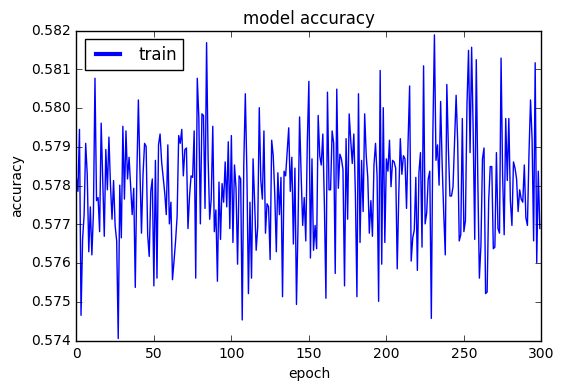In :
# Validating the accuracy and loss of our training set

loss, accuracy = model1.evaluate(X_train, Y_train, verbose=0)
print("Train: accuracy=%f loss=%f" % (accuracy, loss))

Train: accuracy=0.583607 loss=0.893128


Besides the training accuracy, there is also the test accuracy. Testing our model on the training data set is not a fair game. A good testing ground is a completely different set of samples which our model have not already seen. Our testing database consists of 1 milion new poker hands! Let's try them out and see if our model is successful in predicting their class, at least at the same accuracy level as 58% ...

In :
features = ['S1', 'C1', 'S2', 'C2', 'S3', 'C3', 'S4', 'C4', 'S5', 'C5', 'CLASS']

In :
# Checking the success rate on our test set

X_test = tdata.iloc[:,0:10].as_matrix()
y_test = tdata.iloc[:,10].as_matrix()
Y_test = to_categorical(y_test)

loss, accuracy = model1.evaluate(X_test, Y_test, verbose=0)
print("Test: accuracy=%f loss=%f" % (accuracy, loss))

Test: accuracy=0.576027 loss=0.905383

In [ ]:



Test accuracy is 57.6% which is pretty close to the training accuracy (58.1%). This is an encouraging indication that our deep learning model is doing its work as expected. It has been trained on 25,000 samples and gave an exact prediction on a totally different 1 milion samples! This is quite good, so far.

Let's save this model, and proceed to the next one ...

In :
model1.save('model1.h5')


We can get the predictions vectors of our test set in the following way:

In :
y_pred = model1.predict_classes(X_test, verbose=0)


And then count the number of failures

In :
np.count_nonzero(y_pred - y_test)

Out:
423973

We have 423,973 false predictions. We can save them in a numpy array and analyze them

In :
false_preds = [(x,y,p) for (x,y,p) in zip(X_test, y_test, y_pred) if y != p]


Just from looking at the first 20 false predictions we see that our model identified "One Pair" hands with "Nothing in hand", which are very close classes.

In :
false_preds[0:20]

Out:
[(array([ 3, 12,  3,  2,  3, 11,  4,  5,  2,  5], dtype=int64), 1, 0),
(array([1, 9, 4, 6, 1, 4, 3, 2, 3, 9], dtype=int64), 1, 0),
(array([ 1,  4,  3, 13,  2, 13,  2,  1,  3,  6], dtype=int64), 1, 0),
(array([ 4,  7,  3, 12,  1, 13,  1,  9,  2,  6], dtype=int64), 0, 1),
(array([3, 8, 2, 7, 1, 9, 3, 6, 2, 3], dtype=int64), 0, 1),
(array([ 4,  2,  4, 12,  2, 12,  2,  7,  3, 10], dtype=int64), 1, 0),
(array([ 2,  5,  3,  1,  3, 13,  4, 13,  3,  8], dtype=int64), 1, 0),
(array([ 3,  4,  2,  1,  3, 10,  1,  8,  4,  1], dtype=int64), 1, 0),
(array([ 4, 10,  2,  5,  4,  8,  1,  6,  2, 13], dtype=int64), 0, 1),
(array([4, 8, 1, 3, 2, 3, 2, 2, 2, 8], dtype=int64), 2, 1),
(array([1, 4, 4, 5, 4, 3, 1, 8, 4, 1], dtype=int64), 0, 1),
(array([ 1,  7,  4, 13,  1,  5,  1, 13,  3,  3], dtype=int64), 1, 0),
(array([1, 9, 1, 6, 4, 5, 3, 5, 1, 5], dtype=int64), 3, 1),
(array([ 1, 12,  2, 10,  1,  6,  2, 13,  4,  5], dtype=int64), 0, 1),
(array([ 1, 10,  1,  6,  3, 13,  3, 11,  3,  9], dtype=int64), 0, 1),
(array([ 2, 13,  4,  7,  3, 11,  3, 10,  3,  9], dtype=int64), 0, 1),
(array([1, 8, 1, 3, 4, 2, 2, 7, 1, 4], dtype=int64), 0, 1),
(array([1, 1, 4, 5, 4, 1, 3, 7, 4, 4], dtype=int64), 1, 3),
(array([ 2,  7,  3,  7,  4,  4,  1, 10,  1, 13], dtype=int64), 1, 0),
(array([ 2,  2,  4,  3,  4,  8,  1,  2,  3, 13], dtype=int64), 1, 0)]

## Second Keras Model¶

So instead of trying to improve model1 by adding more epochs or tuning activity and optimization, lets build a new model with one extra hidden layer and with more neurons at each layer

In :
model2 = Sequential()

fmon = FitMonitor(thresh=0.03, minacc=0.99, filename="model1.h5")
h = model2.fit(
X_train,
Y_train,
batch_size=32,
nb_epoch=200,
shuffle=True,
verbose=0,
callbacks = [fmon]
)

show_scores(model2, h, X_train, Y_train, X_test, Y_test)

Train begin: 2016-11-20 16:50:05
batch_size = 32
do_validation = False
metrics = ['loss', 'acc']
nb_epoch = 200
nb_sample = 25010
verbose = 0
.......... 10% epoch=20 acc=0.623031 loss=0.854773 max_acc=0.623031 max_val_acc=-1.000000
.......... 20% epoch=40 acc=0.730108 loss=0.677260 max_acc=0.730108 max_val_acc=-1.000000
.......... 30% epoch=60 acc=0.807997 loss=0.513970 max_acc=0.807997 max_val_acc=-1.000000
.......... 40% epoch=80 acc=0.845422 loss=0.411315 max_acc=0.845422 max_val_acc=-1.000000
.......... 50% epoch=100 acc=0.860016 loss=0.370633 max_acc=0.862655 max_val_acc=-1.000000
.......... 60% epoch=120 acc=0.871411 loss=0.338894 max_acc=0.872131 max_val_acc=-1.000000
.......... 70% epoch=140 acc=0.883926 loss=0.311815 max_acc=0.888685 max_val_acc=-1.000000
.......... 80% epoch=160 acc=0.901919 loss=0.267987 max_acc=0.906837 max_val_acc=-1.000000
.......... 90% epoch=180 acc=0.941263 loss=0.182523 max_acc=0.941263 max_val_acc=-1.000000
......... 99% epoch=199 acc=0.942143 loss=0.172708
Train end: 2016-11-20 16:53:32
Total run time: 206.87 seconds
max_acc = 0.958497  epoc = 198
max_val_acc = -1.000000  epoc = -1
No checkpoint model found.
Saving the last state: model1.h5
Training: accuracy   = 0.973531 loss = 0.114517
Validation: accuracy = 0.968519 loss = 0.125479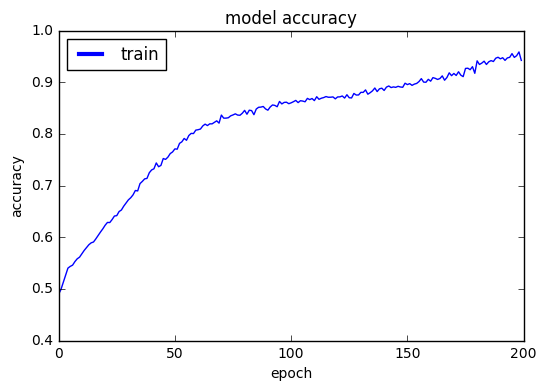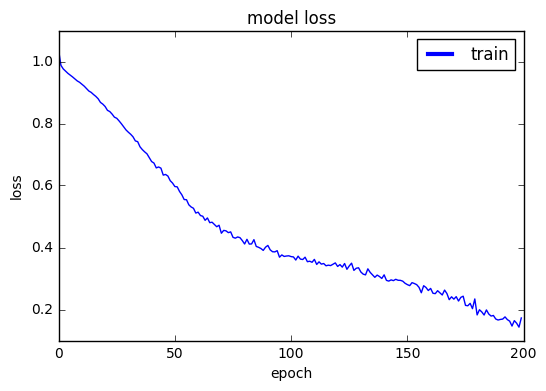This is progressing pretty well: 97.3% accuracy level on our second simple neural network. The model accuracy graph suggests that we get higher accuracy by adding more epochs. Worth trying as an exercise.

Lets see if we get a similar precision rate on our large test set

In :
loss, accuracy = model2.evaluate(X_test, Y_test, verbose=0)
print("Test: accuracy=%f loss=%f" % (accuracy, loss))

Test: accuracy=0.968519 loss=0.125479


This is pretty close and is a strong indication to our model resiliency. Lets sample the false predictions and see what our model has missed:

In :
y_pred = model2.predict_classes(X_test, verbose=1)
false_preds = [(x,y,p) for (x,y,p) in zip(X_test, y_test, y_pred) if y != p]

 998912/1000000 [============================>.] - ETA: 0s
In :
# How many false predictions do we have?
len(false_preds)

Out:
31481
In :
# Lets take a look at 20 samples
[(a, a) for a in false_preds[0:20]]

Out:
[(0, 1),
(0, 1),
(0, 1),
(2, 1),
(0, 1),
(5, 0),
(4, 0),
(2, 1),
(0, 1),
(1, 2),
(2, 3),
(0, 1),
(1, 2),
(2, 3),
(0, 1),
(3, 2),
(3, 2),
(0, 1),
(2, 1),
(1, 0)]

We see that there are stiil mismatches between "one pair" and "Nothing at hand", but now we also see mismatches between "One pair" and "Two pairs", and more ... we can continue and check more samples, but you get the idea. Let's save this model, and proceed to the next one ...

In [ ]:
model2.save("model2.h5")


## Third Keras Model¶

We will use two hidden layers, with 400 neurons each, and run 500 epochs.

In :
model3 = Sequential()

fmon = FitMonitor(thresh=0.03, minacc=0.996, filename="model3.h5")
h = model3.fit(
X_train,
Y_train,
batch_size=32,
nb_epoch=100,
shuffle=True,
verbose=0,
callbacks = [fmon]
)

show_scores(model3, h, X_train, Y_train, X_test, Y_test)

Train begin: 2016-11-20 22:07:30
batch_size = 32
do_validation = False
metrics = ['loss', 'acc']
nb_epoch = 100
nb_sample = 25010
verbose = 0
.......... 10% epoch=10 acc=0.642023 loss=0.809826 max_acc=0.642023 max_val_acc=-1.000000
.......... 20% epoch=20 acc=0.867733 loss=0.343590 max_acc=0.867733 max_val_acc=-1.000000
.......... 30% epoch=30 acc=0.969852 loss=0.116156 max_acc=0.979888 max_val_acc=-1.000000
.......... 40% epoch=40 acc=0.983167 loss=0.066998 max_acc=0.995722 max_val_acc=-1.000000
.......... 50% epoch=50 acc=0.986246 loss=0.053706 max_acc=0.996801 max_val_acc=-1.000000
.......... 60% epoch=60 acc=0.984526 loss=0.054920 max_acc=0.997161 max_val_acc=-1.000000
.......... 70% epoch=70 acc=0.997681 loss=0.009635 max_acc=0.997681 max_val_acc=-1.000000
.......... 80% epoch=80 acc=0.991204 loss=0.029100 max_acc=0.997801 max_val_acc=-1.000000
.......... 90% epoch=90 acc=0.985606 loss=0.057639 max_acc=0.997841 max_val_acc=-1.000000
......... 99% epoch=99 acc=0.976929 loss=0.079074
Train end: 2016-11-20 22:09:54
Total run time: 144.09 seconds
max_acc = 0.997841  epoc = 81
max_val_acc = -1.000000  epoc = -1
No checkpoint model found.
Saving the last state: model3.h5
Training: accuracy   = 0.989524 loss = 0.029236
Validation: accuracy = 0.987381 loss = 0.052444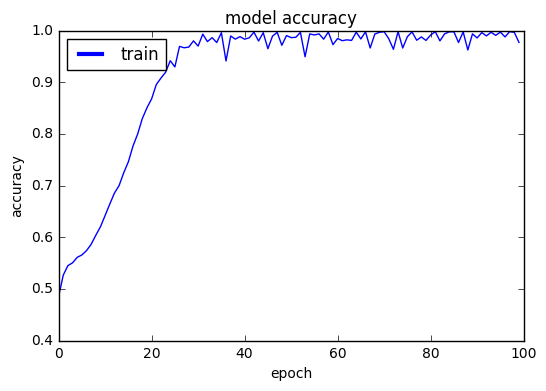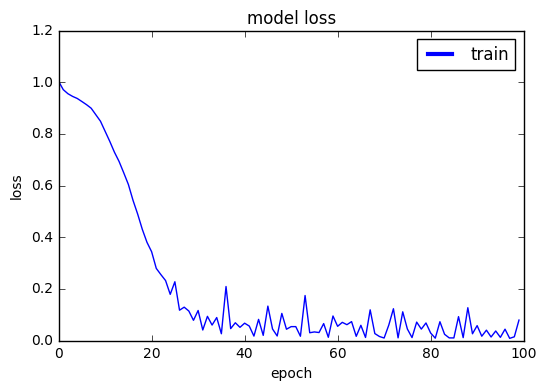In :
# Validating the accuracy and loss of our test set

loss, accuracy = model3.evaluate(X_test, Y_test, verbose=0)
print("Test: accuracy=%f loss=%f" % (accuracy, loss))

Test: accuracy=0.987381 loss=0.052444


Adding more neurons and one more hidden layer did help to get 98.7% accuracy level without really hard work from our part. All we did is guess a few numbers and parameters (very easy to do) and Keras wonderfully produced an efficient clean working model.

It took less that 20 minutes to build this network, and it potentially saves us one month programmer work (are we approaching the end of human programmers? ;-).

From the model accuracy graph it does not look like we can do better by running more epochs. Let's save this model, and proceed to the next one ...

In :
model3.save("model3.h5")


## Fourth Keras Model¶

This time we'll throw a lot of neuron on each layer. This is however not wise or desired in most cases. Large neural networks are prone to be slow and consume much more memory than small ones. This is however a matter of trade-offs and we're still experimenting. Sometimes high precision is worth the extra weight.

In :
model4 = Sequential()

fmon = FitMonitor(thresh=0.03, minacc=0.996, filename="model3.h5")
h = model4.fit(
X_train,
Y_train,
batch_size=32,
nb_epoch=200,
shuffle=True,
verbose=0,
callbacks = [fmon]
)

show_scores(model3, h, X_train, Y_train, X_test, Y_test)

Train begin: 2016-11-20 23:55:51
batch_size = 32
do_validation = False
metrics = ['loss', 'acc']
nb_epoch = 200
nb_sample = 25010
verbose = 0
.......... 10% epoch=20 acc=0.892163 loss=0.290673 max_acc=0.892163 max_val_acc=-1.000000
.......... 20% epoch=40 acc=0.977129 loss=0.079928 max_acc=0.995122 max_val_acc=-1.000000
.......... 30% epoch=60 acc=0.973411 loss=0.102540 max_acc=0.997521 max_val_acc=-1.000000
.......... 40% epoch=80 acc=0.990804 loss=0.037588 max_acc=0.997641 max_val_acc=-1.000000
.......... 50% epoch=100 acc=0.996841 loss=0.014256 max_acc=0.997921 max_val_acc=-1.000000
.......... 60% epoch=120 acc=0.998001 loss=0.008359 max_acc=0.998001 max_val_acc=-1.000000
.......... 70% epoch=140 acc=0.998161 loss=0.007679 max_acc=0.998161 max_val_acc=-1.000000
.......... 80% epoch=160 acc=0.996961 loss=0.013251 max_acc=0.998281 max_val_acc=-1.000000
.......... 90% epoch=180 acc=0.996841 loss=0.013407 max_acc=0.998281 max_val_acc=-1.000000
......... 99% epoch=199 acc=0.998121 loss=0.007230
Train end: 2016-11-21 00:17:34
Total run time: 1302.54 seconds
max_acc = 0.998361  epoc = 189
max_val_acc = -1.000000  epoc = -1
No checkpoint model found.
Saving the last state: model3.h5
Training: accuracy   = 0.998201 loss = 0.008108
Validation: accuracy = 0.996290 loss = 0.037844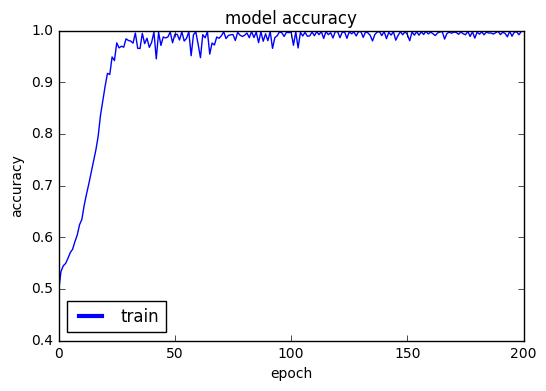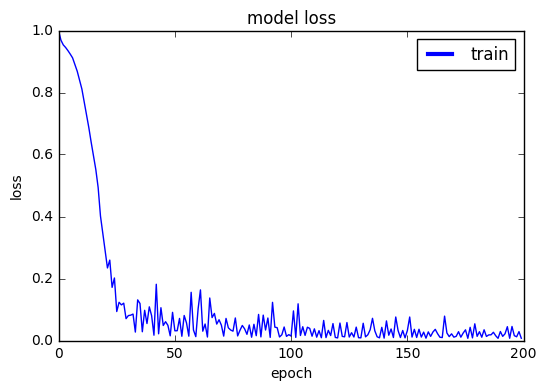In :
# Validating the accuracy and loss of our test set

loss, accuracy = model4.evaluate(X_test, Y_test, verbose=0)
print("Test: accuracy=%f loss=%f" % (accuracy, loss))

Test: accuracy=0.996290 loss=0.037844


Looks like our best achievment so far: 99.8% training accuracy and 99.6% validation accuracy.

Now that you've seen enough examples, you can get creative and try new models. Take into account that Keras offers plenty of activation functions, optimizers, and layer types that we haven't touched at all. So there are literally infinitely many combinations that you can try. As this is planned as a course unit, here is an idea for a project challenge which is also a kind of fun competition to try. I haven't tried it mysel though (I may be wrong with this ambition...), but be glad to see a neat solution if it exists.

## Couse Project Challenge¶

Find a minimal neural network which adhers to the following requirements:

1. Produces at least 99.99% accuracy on the training and testing data sets
2. Number of deep layers does not exceed 8
3. Number of nuerons at each layer does not exceed 200
4. Number of training epochs does not exceed 10000
5. You're not allowed to use any test sample for training! (not even one instance ;-)
6. You will have to submit a full model definition from the ground up: layers, compilation, and training methods

The solution with the smallest number of neurons will receive the highest grade, and the remaining solutions will receive smaller grades relative to their ranking compared to the best solution.